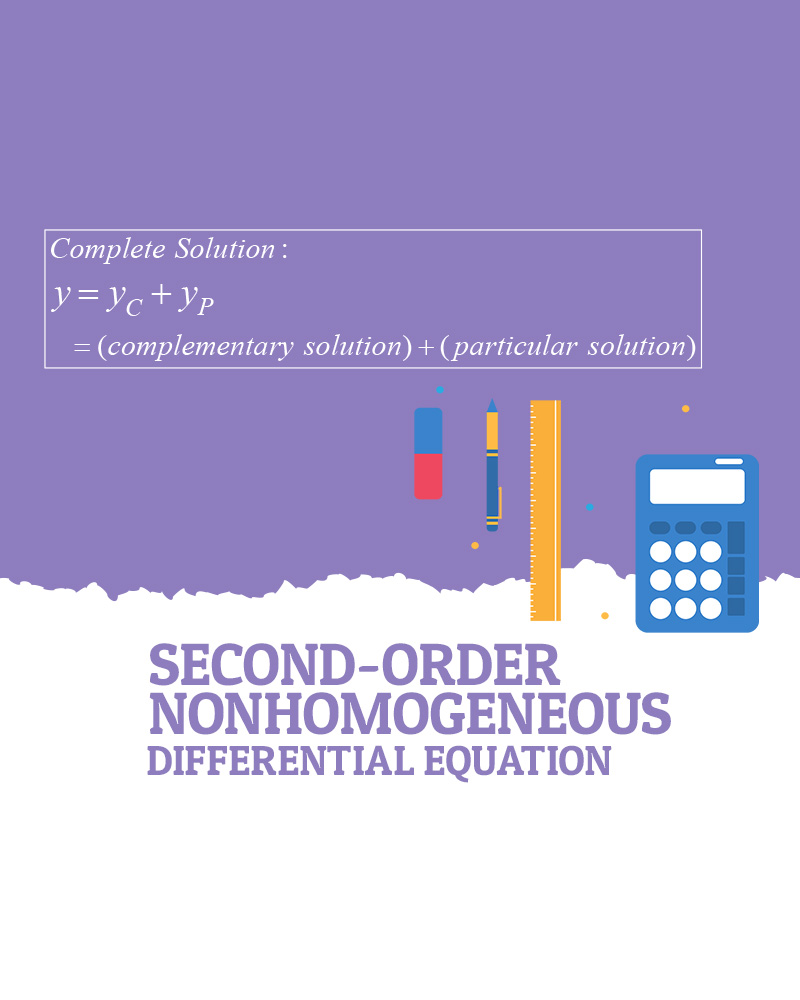# 2nd order Nonhomogeneous Differential Equation

Before looking at the example, you need to know the solution formula for second-order differential equations ay”+ by’ +cy = f(x) as shown.  Notice that there are two parts, y-sub C and y-sub P in the complete solution.

One part, y-sub C is solving a homogeneous differential equation.  Y-sub C is often called a complementary solution.